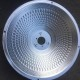# Fitting Calculation of Non-circular Curve Nodes in Precision Parts Machining

At present, most precision parts processing machine tools do not have the interpolation function of non-circular curves. Therefore, when processing these curves, the method of fitting straight or circular segments is usually used. In the manual programming process, the commonly used fitting There are several calculation methods such as equal interval method, equal interpolation segment method and three-point fixed circle method, so what kind of fitting calculation methods do they have? Here is an explanation for you:

(1) Equal spacing method:

``In the direction of a coordinate axis, the total increment of the fitted contour (if in the polar coordinate system, the total increment of the corner or radial coordinate) is divided into equal parts, and then the coordinate value of the set node is calculated The method is called the equidistant method.``

In the actual programming process, this method is easy to control the nodes of the non-circular curve, so this method is commonly used in the macro program (or parameter) of the precision part processing.

(2) Equal interpolation segment method:

When the chord lengths of two adjacent nodes are set to be equal, the method of calculating the coordinate values ​​of the nodes for the modified contour curve is called equal interpolation segment method.

(3) Three-point circle method:

In the programming method of precision parts processing, this is a method of fitting three points on a non-circular curve with an arc (including the center and radius).Author: Chris Na